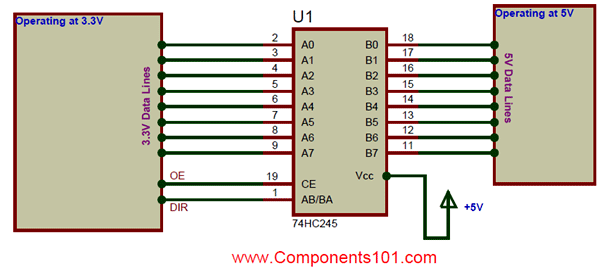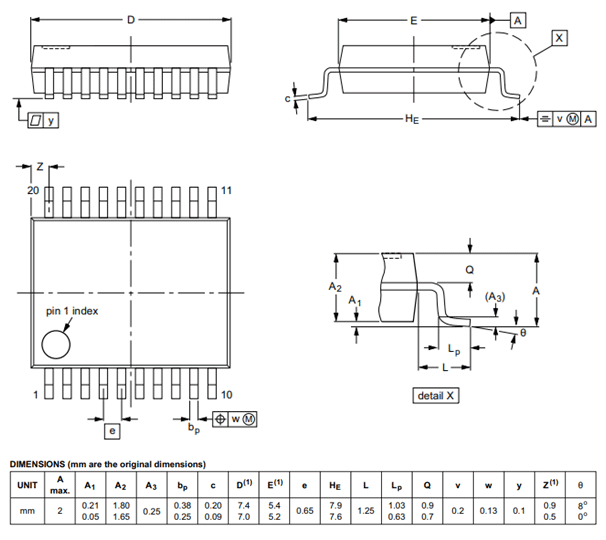# 74HC245 8-bit Transceiver IC

### 74HC245Pin Configuration

 Pin Number Pin Name Description 1 Direction Control (DIR) This pin decides the direction of the Data. 2 to 9 Data Input/output (A0-A7) These 8 pins can be used as 8-bit Input or 8-bit Output pins based on the state of DIR pin 10 Ground (GND) Connected to the ground of the system 11 to 18 Data Input/output (B0-B7) These 8 pins can be used as 8-bit Input or 8-bit Output pins based on the state of DIR pin 19 Output Enable (OE) Active Low pin – Used to Enable/Disable Input 20 Supply Voltage (Vcc) Differential Analog input +. Connect to ADC input

### Features

• 8-bit transceiver with 3-state
• Supply Voltage: 2V to 6V
• Output Voltage is equal to Vcc
• Minimum Input Voltage: 0.8V
• Maximum Input Voltage: 4.2V
• Minimum Output Voltage: 1.9V
• Maximum Output Voltage: 5.4V
• Output Current: 35mA
• Off-State Output Current: 10uA
• Non-Inverting, hence easy to interface
• Available in 20-pin SO20, SSOP20, TSSOP20 and DHVQFN20 packages

Note: Complete Technical Details can be found in the 74HC245 datasheet given at the end of this page.

SN74S245

### Alternative Transceiver IC

MCP2551, MCP2515, SN7516

### Where to use a 74HC245 IC

The 74HC245 is an 8-bit transceiver IC with 3-state output. Meaning it has two set of pins (A0-A7 and B0-B7), in which each set has 8 pins. Out of these two sets one set can be used as input and the other set can be used as output. Since the IC is a transceiver IC, Data can flow from input bus to output bus and also from output bus to input bus, by simply toggling the Direction pin (DIR) pin as required. It also has a decent output current of 35mA and hence can be used to drive nominal loads.

So if you are looking for an IC to act as a buffer or as a transceiver for your address or data bus. Or if you are looking for an IC to act as a 8-bit logic level converter then 74HC245 IC might be the right choice for you. It is also possible to use this IC for establishing communication protocol buses (SPI, IIC, etc.) between two different MCU/MPU with different operating voltages since the IC has high speed buffer. The 3 State at which 74HC245 IC can operate is shown in the table, the pins OE and DIR can be used as follows to set the state.### How to use the 74HC245 IC

74HC245 IC has a vast application; however for simplicity let us consider we are using the IC to act as logic level converter. Many hardware like Raspberry Pi, MSP430 operates with a voltage of 3.3V, but other sensors or IC’s like Ultrasonic sensor, TFT LCD displays etc operate in 5V. So in these cases we have to shift one set of operating voltage (3.3V) to another set (5V). In these cases we employ a logic level converter; the simplest logic level converter is a potential divider. But it is not efficient for high speed operation or for bi-directional communication buses. Hence in those cases a bi-directional transceiver IC like 74HC245 seems to be an ideal choice.A general circuit diagram using IC 74HC245 as a logic level converter is shown above. Here we are converting the 3.3V data lines to 5V data Lines. The Data can be made to flow in both the directions by controlling the DIR (AB/BA) as shown in the table above. Also we can reduce the input leakage current by disabling the inputs by using the OE pin as shown in the table above. The output voltage will always be equal to the Vcc voltage, so here since we are converting the 3.3V to 5V, we should power the Vcc pin with +5V as shown above.

### Applications

• Data bus transceivers
• Logic level converters
• Driver IC for communication protocols

### 2D – Model (SSOP20) and DimensionsComponent Datasheet

## Related Post

### Join 20K+subscribers

We will never spam you.

* indicates required

Be a part of our ever growing community.